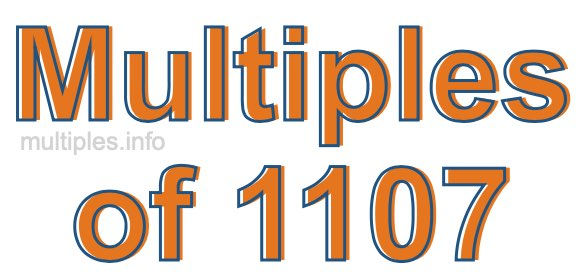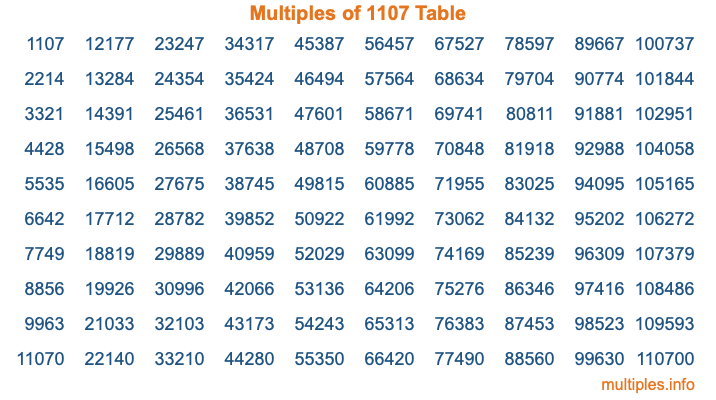Multiples of 1107Welcome to the Multiples of 1107 page. Here we will first teach you everything you will ever need to know about the multiples of 1107, and then give you a study guide summary of everything we taught you to make sure you remember it all. Use this page to look up facts and learn information about the multiples of 1107. This page will make you a multiples of one thousand one hundred seven expert!

Definition of Multiples of 1107
Multiples of 1107 are all the numbers that when divided by 1107 equal an integer. Each of the multiples of 1107 are called a multiple. A multiple of 1107 is created by multiplying 1107 by an integer.

Therefore, to create a list of multiples of 1107, you start with 1 multiplied by 1107, then 2 multiplied by 1107, then 3 multiplied by 1107, and so on for as long as you want. Thus, the list of the first five multiples of 1107 is 1107, 2214, 3321, 4428, and 5535. To see a larger list of multiples of 1107, see the printable image of Multiples of 1107 further down on this page. We also have a category where you can choose any nth multiple of 1107.

Multiples of 1107 Checker
The Multiples of 1107 Checker below checks to see if any number of your choice is a multiple of 1107. In other words, it checks to see if there is any number (integer) that when multiplied by 1107 will equal your number. To do that, we divide your number by 1107. If the the quotient is an integer, then your number is a multiple of 1107.

Is  a multiple of 1107?

Least Common Multiple of 1107 and ...
A Least Common Multiple (LCM) is the lowest multiple that two or more numbers have in common. This is also called the smallest common multiple or lowest common multiple and is useful to know when you are adding our subtracting fractions. Enter one or more numbers below (1107 is already entered) to find the LCM.

Check out our LCM Calculator if you need more details about the Least Common Multiple or if you need the LCM for different numbers for adding and subtraction fractions.

nth Multiple of 1107
As we stated above, 1107 is the first multiple of 1107, 2214 is the second multiple of 1107, 3321 is the third multiple of 1107, and so on. Enter a number below to find the nth multiple of 1107.

th multiple of 1107

Multiples of 1107 vs Factors of 1107
1107 is a multiple of 1107 and a factor of 1107, but that is where the similarities end. All postive multiples of 1107 are 1107 or greater than 1107. All positive factors of 1107 are 1107 or less than 1107.

Below is the beginning list of multiples of 1107 and the factors of 1107 so you can compare:

Multiples of 1107: 1107, 2214, 3321, 4428, 5535, etc.

Factors of 1107: 1, 3, 9, 27, 41, 123, 369, 1107

As you can see, the multiples of 1107 are all the numbers that you can divide by 1107 to get a whole number. The factors of 1107, on the other hand, are all the whole numbers that you can multiply by another whole number to get 1107.

It's also interesting to note that if a number (x) is a factor of 1107, then 1107 will also be a multiple of that number (x).

Multiples of 1107 vs Divisors of 1107
The divisors of 1107 are all the integers that 1107 can be divided by evenly. Below is a list of the divisors of 1107.

Divisors of 1107: 1, 3, 9, 27, 41, 123, 369, 1107

The interesting thing to note here is that if you take any multiple of 1107 and divide it by a divisor of 1107, you will see that the quotient is an integer.

Multiples of 1107 Table
Below is an image of the first 100 multiples of 1107 in a table. The table is in chronological order, column by column. The first column has the first ten multiples of 1107, the second column has the next ten multiples of 1107, and so on.The Multiples of 1107 Table is also referred to as the 1107 Times Table or Times Table of 1107. You are welcome to print out our table for your studies.

Negative Multiples of 1107
Although not often discussed or needed in math, it is worth mentioning that you can make a list of negative multiples of 1107 by multiplying 1107 by -1, then by -2, then by -3, and so on, to get the following list of negative multiples of 1107:

-1107, -2214, -3321, -4428, -5535, etc.

Multiples of 1107 Summary
Below is a summary of important Multiples of 1107 facts that we have discussed on this page. To retain the knowledge on this page, we recommend that you read through the summary and explain to yourself or a study partner why they hold true.

There are an infinite number of multiples of 1107.

A multiple of 1107 divided by 1107 will equal a whole number.

1107 divided by a factor of 1107 equals a divisor of 1107.

The nth multiple of 1107 is n times 1107.

The largest factor of 1107 is equal to the first positive multiple of 1107.

1107 is a multiple of every factor of 1107.

1107 is a multiple of 1107.

A multiple of 1107 divided by a divisor of 1107 equals an integer.

1107 divided by a divisor of 1107 equals a factor of 1107.

Any integer times 1107 will equal a multiple of 1107.

Multiples of a Number
Here you can get the multiples of another number, all with the same attention to detail as we did for multiples of 1107 on this page.

Multiples of
Multiples of 1108
Did you find our page about multiples of one thousand one hundred seven educational? Do you want more knowledge? Check out the multiples of the next number on our list!